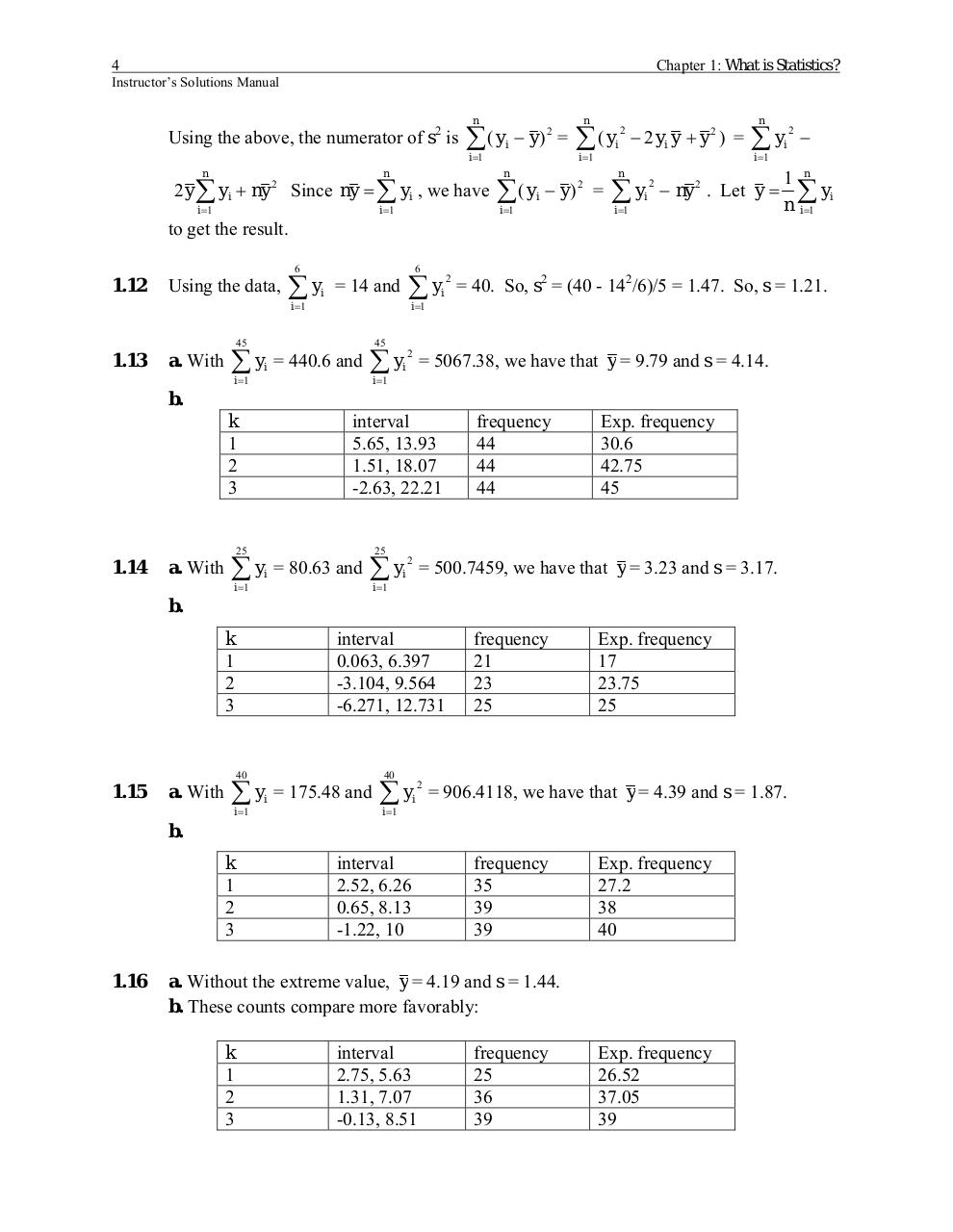# Merged PDF Solutions Manual.pdfPage 1 2 3 456333

#### Text preview

4

Chapter 1: What is Statistics?

Instructor’s Solutions Manual
n

2

Using the above, the numerator of s is

∑( y
i =1

n

n

i =1

i =1

2 y ∑ yi + ny 2 Since ny = ∑ yi , we have

− y) =
2

i

n

n

∑( y
i =1

∑ ( yi − y ) 2 =
i =1

2
i
n

− 2 yi y + y ) =
2

n

∑y
i =1

∑ yi − ny 2 . Let y =
2

i =1

2
i

1 n
∑ yi
n i =1

to get the result.
6

1.12

Using the data,

6

∑ yi = 14 and

∑y

i =1

45

1.13

a. With

∑ yi = 440.6 and
i =1

i =1

45

∑y
i =1

2

2
i

= 40. So, s2 = (40 - 142/6)/5 = 1.47. So, s = 1.21.

= 5067.38, we have that y = 9.79 and s = 4.14.

i

b.

interval
5.65, 13.93
1.51, 18.07
-2.63, 22.21

k
1
2
3
25

1.14

a. With

∑y
i =1

25

i

= 80.63 and

∑y
i =1

2

frequency
44
44
44

Exp. frequency
30.6
42.75
45

= 500.7459, we have that y = 3.23 and s = 3.17.

i

b.

interval
0.063, 6.397
-3.104, 9.564
-6.271, 12.731

k
1
2
3

40

1.15

a. With

∑y
i =1

40

i

= 175.48 and

∑y
i =1

2
i

frequency
21
23
25

Exp. frequency
17
23.75
25

= 906.4118, we have that y = 4.39 and s = 1.87.

b.

k
1
2
3
1.16

interval
2.52, 6.26
0.65, 8.13
-1.22, 10

frequency
35
39
39

Exp. frequency
27.2
38
40

a. Without the extreme value, y = 4.19 and s = 1.44.
b. These counts compare more favorably:

k
1
2
3

interval
2.75, 5.63
1.31, 7.07
-0.13, 8.51

frequency
25
36
39

Exp. frequency
26.52
37.05
39